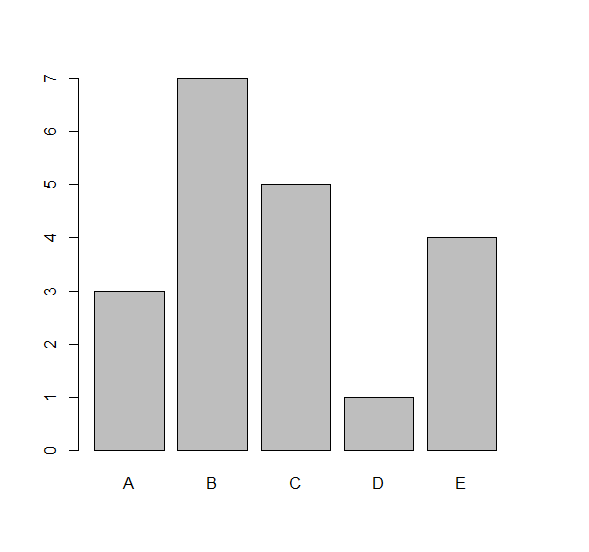# How to deal with hist.default, 'x' must be numeric in R?

The error hist.default, 'x' must be numeric occurs when we pass a non-numerical column or vector to the hist function. If we have a non-numerical column in a data frame or a non-numerical vector and we want to create the histogram of that data can be created with the help of barplot and table function.

Check out the below given Example

to understand how it can be done.

## Example

Following snippet creates a sample data frame −

x<-sample(LETTERS[1:5],20,replace=TRUE)
df<-data.frame(x)
df

The following dataframe is created

   x
1 C
2 B
3 B
4 B
5 E
6 E
7 C
8 A
9 C
10 B
11 D
12 E
13 A
14 C
15 B
16 A
17 C
18 E
19 B
20 B

To create histogram of x with hist function on the above created data frame, add the following code to the above snippet −

x<-sample(LETTERS[1:5],20,replace=TRUE)
df<-data.frame(x)
hist(df$x) To create histogram of x with barplot function on the above created data frame, add the following code to the above snippet − x<-sample(LETTERS[1:5],20,replace=TRUE) df<-data.frame(x) barplot(table(df$x))

## Output

If you execute all the above given snippets as a single program, it generates the following Output −